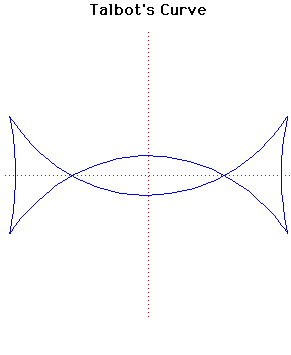# Talbot's CurveParametric Cartesian equation:
x = (a2 + f2sin2(t))cos(t)/a, y = (a2 - 2f2 + f2sin2(t))sin(t)/b

Click below to see one of the Associated curves.

Click THIS LINK to experiment interactively with this curve and its associated curves.

This curve was investigated by Talbot.

Talbot's curve is the negative pedal of an ellipse with respect to its centre. It has four cusps and two nodes provided the square of the eccentricity of the ellipse is greater than 1/2.

JOC/EFR/BS January 1997